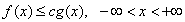ID 772987
Date 12/04/2020
Public

## Acceptance/Rejection

The cumulative distribution functions and their inverses may often be much more complex computationally than the probability density function (for continuous distributions) and the probability mass function (for discrete distributions).Therefore, methods based on the use of density (mass) functions are often more efficient than the inverse transformation method.

Consider a case of continuous probability distribution:

Suppose, you need to generate random numbers x with distribution density f(x). Apart from the variate X, consider the variate Y with the density g(x), which has a fast method of random number generation and the constant c such that.

Then, it is easy to conclude that the following algorithm provides generation of random numbers x with the distribution F(x):

1. Generate a random number y with the distribution density g(x).

2. Generate a random number u (independent of y) that is uniformly distributed over the interval (0, 1).

3. If u≤f(y)/cg(y), accept y as a random number x with the distribution F(x). Otherwise, go back to step 1.

The efficiency of this method depends on the degree of complexity of random number generation with distribution density g(x), computational complexity for the functions f(x) and g(x), as well as on the constant c value. The closer c is to 1, the lower the necessity to reject the generated y.

NOTE:

Since quasi-random sequences are non-random, you should be careful when using quasi-random basic generators with the acceptance/rejection methods.